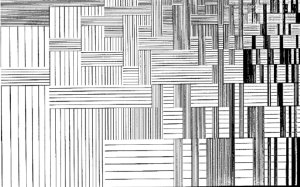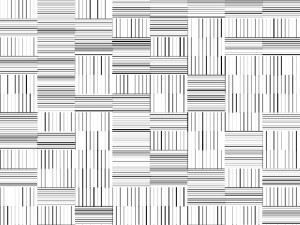# Random Vertical/Horizontal Lines

I find this particular image interesting among all the old computer graphics available:First I tried to imitate the image by creating vertical lines as the background, and then added rectangular shapes covered by horizontal lines. It did not work as I expected: Somehow the vertical and horizontal lines form the shape of checks instead of stitches. Then I tried another way, making vertical or horizontal lines appear randomly. Then the color( 0 to 255) and the stroke( 1 to 3) would also vary randomly. The sad thing is, I could not recreate the image as it is —- larger gaps between the lines towards the lower left , and denser pattern towards the upper right corner. One of the graphics the code generates looks like this:My code looks like this:

```int columnN = 8;
int rowN = 20;

void setup() {
size(800, 600);
background(255);

int horizLine = width/ columnN;
int vertLine = horizLine/2;

for( int i = 0; i < rowN; i++) {
for(int j = 0; j < columnN; j++) {
float horiz_vert = random(0,2);
if( horiz_vert <1) {
// draw vertical lines with random stroke color and weight
for( float k = 0; k < horizLine; k += horizLine/10) {
stroke(random(0,255));
strokeWeight(random(1,3));
line(j*horizLine+k, i*vertLine, j*horizLine+k, i*vertLine+vertLine);
}
}else{
// draw horizontal lines
for( float k = 0; k < vertLine; k += vertLine/10) {
stroke(random(0,255));
strokeWeight(random(1,3));
line(j*horizLine, i*vertLine+k, j*horizLine+ horizLine, i*vertLine+k);
}
}
}
}
}

void draw() {
}

void mousePressed() {
saveFrame( " Random Stitch Pattern. jpg");
}```